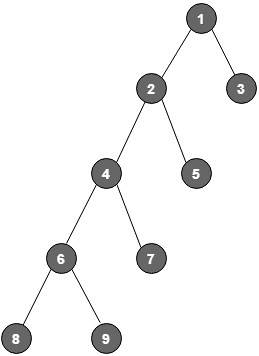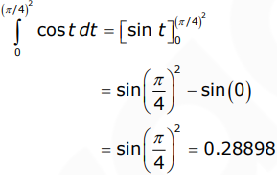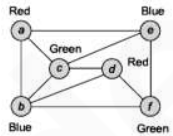# GATE Solved Paper 2017-19 - GATE 2018

• A

3• B

2• C

4• D

5• Option : C
• Explanation :Given, post-order – 8, 9, 6, 7, 4, 5, 2, 3, 1 and in-order – 8, 6, 9, 4, 7, 2, 5, 1, 3
So the height of tree is 4.

• A

0.6944• B

0.1157• C

0.023• D

0.463• Option : C
• Explanation :
Given there are two identical dices, each having 6 faces.
Favorable events for tie = {(1, 1), (2, 2), (3, 3), (4, 4), (5, 5), (6, 6)}
Since two dice are thrown, total sample space will be 6 x 6 = 36

Therefore, P(tie) = 6/36 = 1/6
and Probability of not tie = (1 – 1/6)
To one of them win on third trial, previous two trials should be tie.

= 1/6 * 1/6 * (1 – 1/6)
= 1/36 * 5/6
= 5/216 = 0.023
So, option (C) is correct.

correct to three decimal places (assuming that π = 3.14) is __________.

Note – Numerical Type question

• A

0.389• B

0.289• C

0.829• D

0.428• Option : B
• Explanation :put x2 = t
2xdx = dt or x dx = 1/2 dt
if x = 0 then t = 0 and if x = π/4 then t = (π/4)2Note – Numerical Type question

• A

3• B

4• C

5• D

2• Option : A
• Explanation :
Sub graph is K3 chromatic number is at least 3. We can try for a chromatic number of 3 by using 3 colors, as follows :All vertices with only 3 colors, the chromatic number of this graph is 3.

• A

58• B

57• C

59• D

60• Option : C
• Explanation :
Total number of rows is 214 and time taken to perform one refresh operation is 50 nanoseconds. So, total time taken to perform refresh operation = 214*50 nanoseconds = 819200 nanoseconds = 0.819200 milliseconds.
Refresh period is 2 milliseconds. So, time spent in refresh period in percentage = (0.819200 milliseconds) / (2 milliseconds) = 0.4096 = 40.96%
Hence, time spent in read/write operation = 100% – 40.96% = 59.04% = 59 (in percentage and rounded to the closet integer).
So, answer is 59.
Related Quiz.
GATE 2018